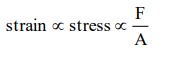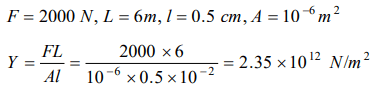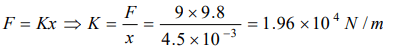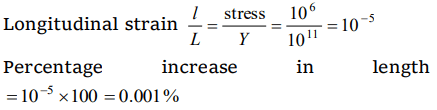## Mechanical Properties of Solids Questions and Answers Part-4

1. Two wires of copper having the length in the ratio 4 : 1 and their radii ratio as 1 : 4 are stretched by the same force. The ratio of longitudinal strain in the two will be
a) 1 : 16
b) 16 : 1
c) 1 : 64
d) 64 : 1

Explanation:2. A weight of 200 kg is suspended by vertical wire of length 600.5 cm. The area of cross-section of wire is $1mm^{2}$ . When the load is removed, the wire contracts by 0.5 cm. The Young's modulus of the material of wire will be
a) $2.35\times10^{12} N\diagup m^{2}$
b) $1.35\times10^{10} N\diagup m^{2}$
c) $13.5\times10^{11} N\diagup m^{2}$
d) $23.5\times10^{9} N\diagup m^{2}$

Explanation:3. If a load of 9 kg is suspended on a wire, the increase in length is 4.5 mm. The force constant of the wire is
a) $0.49\times10^{4} N\diagup m$
b) $1.96\times10^{4} N\diagup m$
c) $4.9\times10^{4} N\diagup m$
d) $0.196\times10^{4} N\diagup m$

Explanation:4.The ratio of diameters of two wires of same material is n : 1. The length of wires are 4 m each. On applying the same load, the increase in length of thin wire will be
a) $n^{2} times$
b) n times
c) 2n times
d) None of the above

Explanation:5. Longitudinal stress of $1 kg / mm^{2}$  is applied on a wire. The percentage increase in length is $\left(Y=10^{11} N\diagup m^{2}\right)$
a) 0.002
b) 0.001
c) 0.003
d) 0.01

Explanation:6. A steel wire is stretched with a definite load. If the Young's modulus of the wire is Y. For decreasing the value of Y
a) Radius is to be decreased
c) Length is to be increased
d) None of the above

Explanation: It is the specific property of a particular metal at a given temperature which can be changed only by temperature variations.

7. The interatomic distance for a metal is $3\times10^{-10} m$   . If the interatomic force constant is $3.6\times10^{-9} N\diagup \dot{A}$    , then the Young's modulus in $N\diagup m^{2}$  will be
a) $1.2\times10^{11}$
b) $4.2\times10^{11}$
c) $10.8\times10^{-19}$
d) $2.4\times10^{10}$

Explanation:8. Two identical wires of rubber and iron are stretched by the same weight, then the number of atoms in the iron wire will be
a) Equal to that of rubber
b) Less than that of the rubber
c) More than that of the rubber
d) None of the above

Explanation: More than that of the rubber

9. The force constant of a wire does not depend on
a) Nature of the material
c) Length of the wire
d) None of the above